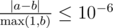# CF605C Freelancer's Dreams

• 10通过
• 40提交
• 题目来源
• 评测方式 RemoteJudge
• 标签 凸包 向量
• 难度 省选/NOI-
• 时空限制 2000ms / 256MB
• 提示：收藏到任务计划后，可在首页查看。

## 题意翻译

有 $n$ 份工作，完成 $1$ 个单位时间的第 $i$ 项工作会获得 $a_i$ 和 $b_i$ 两项属性值。 工作的单位时间数可以不是整数 。你需要在尽量短的时间内使 $\sum a\ge p,\sum_b\ge q$ 。

$1\le n\le 10^5,1\le a_i,b_i,p,q\le 10^6$

为了防止精度问题，若你的答案为 $a$ ，而标程给出的答案为 $b$ ，你的答案会被认为是正确的当且仅当 $\frac {|a-b|}{\max(1,b)}\le 10^{-6}$ 。

## 题目描述

Mikhail the Freelancer dreams of two things: to become a cool programmer and to buy a flat in Moscow. To become a cool programmer, he needs at least $p$ experience points, and a desired flat in Moscow costs $q$ dollars. Mikhail is determined to follow his dreams and registered at a freelance site.

He has suggestions to work on $n$ distinct projects. Mikhail has already evaluated that the participation in the $i$ -th project will increase his experience by $a_{i}$ per day and bring $b_{i}$ dollars per day. As freelance work implies flexible working hours, Mikhail is free to stop working on one project at any time and start working on another project. Doing so, he receives the respective share of experience and money. Mikhail is only trying to become a cool programmer, so he is able to work only on one project at any moment of time.

Find the real value, equal to the minimum number of days Mikhail needs to make his dream come true.

For example, suppose Mikhail is suggested to work on three projects and $a_{1}=6$ , $b_{1}=2$ , $a_{2}=1$ , $b_{2}=3$ , $a_{3}=2$ , $b_{3}=6$ . Also, $p=20$ and $q=20$ . In order to achieve his aims Mikhail has to work for $2.5$ days on both first and third projects. Indeed, $a_{1}·2.5+a_{2}·0+a_{3}·2.5=6·2.5+1·0+2·2.5=20$ and $b_{1}·2.5+b_{2}·0+b_{3}·2.5=2·2.5+3·0+6·2.5=20$ .

## 输入输出格式

输入格式：

The first line of the input contains three integers $n$ , $p$ and $q$ ( $1<=n<=100000,1<=p,q<=1000000$ ) — the number of projects and the required number of experience and money.

Each of the next $n$ lines contains two integers $a_{i}$ and $b_{i}$ ( $1<=a_{i},b_{i}<=1000000$ ) — the daily increase in experience and daily income for working on the $i$ -th project.

输出格式：

Print a real value — the minimum number of days Mikhail needs to get the required amount of experience and money. Your answer will be considered correct if its absolute or relative error does not exceed $10^{-6}$ .

Namely: let's assume that your answer is $a$ , and the answer of the jury is $b$ . The checker program will consider your answer correct, if.

## 输入输出样例

输入样例#1： 复制
3 20 20
6 2
1 3
2 6

输出样例#1： 复制
5.000000000000000

输入样例#2： 复制
4 1 1
2 3
3 2
2 3
3 2

输出样例#2： 复制
0.400000000000000


## 说明

First sample corresponds to the example in the problem statement.

提示
标程仅供做题后或实在无思路时参考。
请自觉、自律地使用该功能并请对自己的学习负责。
如果发现恶意抄袭标程，将按照I类违反进行处理。